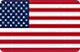# Risk and return calculator

• Result
• 0.382Drawn-down
• 0.618Drawn-down
• First target
• Second target
• Third target
• Risk Manage
• Return
• Rate
Attention! If the risk return rate is less than 2, we do not recommend you to participate and trade.

# Position Size Calculator

When day trading foreign exchange (forex) rates, position size, or transaction size in units, is more essential than entry and exit points. This means you'll take on too much or not enough risk depending on how big or small your trades will be.
Using a free Forex position size calculator, you may precisely assess your risk exposure by determining the number of units or lots that your trade will include. Every major currency pair and cross is supported. Input values are sparse, but the ability to fine-tune it to your individual requirements is there.
To get started, simply enter your information in the fields provided and hit the "Calculate" button.
The number of lots you buy or sell, as well as the type and size of lots, significantly affect the size of your position:
1,000 units of a currency make up a “micro lot”
10,000 units of currency make up a “mini lot”
100,000 units of currency make up a “standard lot”
The ideal position size can be calculated through the following formula:
Pips at risk * pip value * lots traded = amount at risk
Consider this example, if you have a \$10,000 account and risk 1% of your account on each trade, a \$10,000 account with \$10,000 in total. Thus, the maximum amount you can risk per trade is \$100. At \$1.3051, you decide to purchase the EUR/USD pair and set a stop loss at \$1.3041. To put it another way, you're risking \$1.3041 (\$1.3051 – \$1.3041 = \$0.0001). Each pip movement is worth \$1 because you've been trading mini lots.
If you plug those numbers in the formula, you get:
10 * \$1 * lots traded = \$100
If you divide both sides of the equation by \$10, you arrive at:
10 mini lots are equal to one standard lot, you could buy either 10 minis or one standard.
Another example, you're trading mini lots of the EUR/GBP and you decide to buy at \$0.9804 and place a stop loss at \$0.9794. That again is 10 pips of risk.
10 * \$1.22 * lots traded = \$100
The \$1.22 value comes from the above conversion formula and this number varies in light of the current exchange rate between the US dollar and the British pound. If you divide both sides of the equation by \$12.20, you arrive at:
As a result, your position size should be eight mini lots and one micro lot. Using this calculation formula and the 1% rule, you'll be able to accurately determine the lot and position sizes in your forex trading journey.
• Result
• Risk Manage
• Return
• Rate
• 0.382Drawn-down
• Result
• Risk Manage
• Return
• Rate
• 0.618Drawn-down
• Result
• Risk Manage
• Return
• Rate
• First target
• Result
• Risk Manage
• Return
• Rate
• Second target
• Result
• Risk Manage
• Return
• Rate
• Third target

# Position Size Calculator

When day trading foreign exchange (forex) rates, position size, or transaction size in units, is more essential than entry and exit points. This means you'll take on too much or not enough risk depending on how big or small your trades will be.
Using a free Forex position size calculator, you may precisely assess your risk exposure by determining the number of units or lots that your trade will include. Every major currency pair and cross is supported. Input values are sparse, but the ability to fine-tune it to your individual requirements is there.
To get started, simply enter your information in the fields provided and hit the "Calculate" button.
The number of lots you buy or sell, as well as the type and size of lots, significantly affect the size of your position:
1,000 units of a currency make up a “micro lot”
10,000 units of currency make up a “mini lot”
100,000 units of currency make up a “standard lot”
The ideal position size can be calculated through the following formula:
Pips at risk * pip value * lots traded = amount at risk
Consider this example, if you have a \$10,000 account and risk 1% of your account on each trade, a \$10,000 account with \$10,000 in total. Thus, the maximum amount you can risk per trade is \$100. At \$1.3051, you decide to purchase the EUR/USD pair and set a stop loss at \$1.3041. To put it another way, you're risking \$1.3041 (\$1.3051 – \$1.3041 = \$0.0001). Each pip movement is worth \$1 because you've been trading mini lots.
If you plug those numbers in the formula, you get:
10 * \$1 * lots traded = \$100
If you divide both sides of the equation by \$10, you arrive at:
10 mini lots are equal to one standard lot, you could buy either 10 minis or one standard.
Another example, you're trading mini lots of the EUR/GBP and you decide to buy at \$0.9804 and place a stop loss at \$0.9794. That again is 10 pips of risk.
10 * \$1.22 * lots traded = \$100
The \$1.22 value comes from the above conversion formula and this number varies in light of the current exchange rate between the US dollar and the British pound. If you divide both sides of the equation by \$12.20, you arrive at:United States